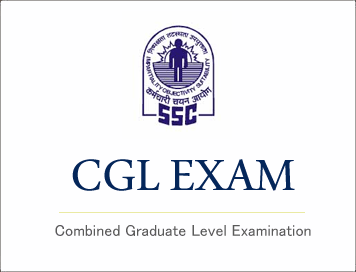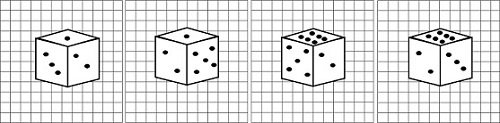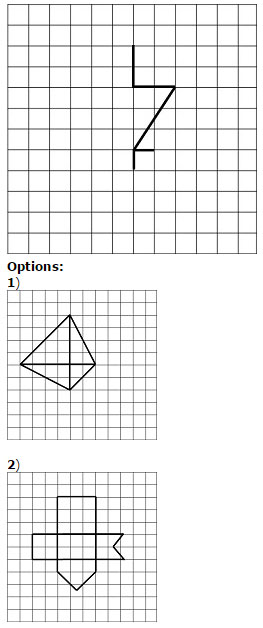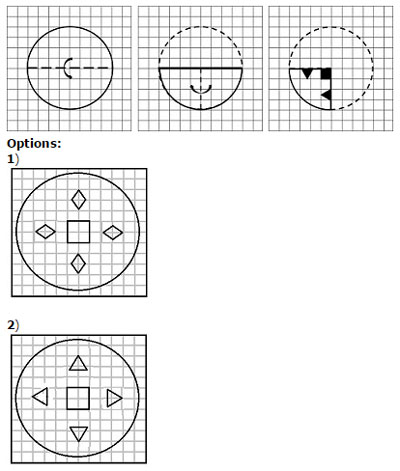# SSC CGL (Tier - 1) Online Exam Paper - 2016 "held on 8 September 2016" Evening Shift (Reasoning)## SSC CGL (Tier - 1) Online Exam Paper - 2016 "held on 8 September 2016" Evening Shift (Reasoning)

EXAM DATE : 8-September-2016
EXAM START TIME :
16:15:00
EXAM NAME :
SSC Examination 2016

Question 1.Select the related word/letters/numbers from the given alternatives:
Air:Bird::Water: ?

Options:

1) Fish
2) Swim
3) Wash
4) Drink

Question 2.Select the related word/letters/numbers from the given alternatives:
ACEG:BDFH::IKMO: ?

Options:

1) IJKL
2) JLNP
3) KLMN
4) HIJK

Question 3.Select the related word/letters/numbers from the given alternatives:
12:20::30: ?

Options:

1) 15
2) 35
3) 42
4) 48

Question 4.
For the following questions
Find the odd word/letters/number pair from the given alternatives.
(A) Neurologist (B) Dentist (C) Architect (D) Paediatrician

Options:

1) A
2) B
3) C
4) D

Question 5.
For the following questions
Find the odd word/letters/number pair from the given alternatives.

Options:

1) A
2) B
3) C
4) D

Question 6.
For the following questions
Find the odd word/letters/number pair from the given alternatives.

Options:

1) A
2) B
3) C
4) D

Question 7.Arrange the following words as per order in the dictionary.
1. Syringe 2. Syphilis 3. Syncretism 4. Synarchy 5. Submerge

Options:

1) 4,5,2,3,1
2) 5,4,3,2,1
3) 5,3,4,1,2
4) 4,1,2,3,5

Question 8.A series is given, with one term missing. Choose the correct alternative from the given ones that will complete the series.
WYV, ? , IKH, BDA

Options:

1) OPR
2) ROP
3) PRO
4) OQH

Question 9.Which number will complete the series?
6 , 8 , 17 , 19 , 28 , 30 , ?

Options:

1) 32
2) 37
3) 38
4) 39

Question 10.A is B's brother, C is A's mother, D is C's father, F is A's son. How is A related to F's child?

Options:

1) Aunt
2) Cousin
3) Nephew
4) Grandfather

## Study Kit for SSC CGL EXAM

Question 11.Seven boys A,B,C,D,E,F and G are standing in a line.

(i) G is between A and E
(ii) F and A have one boy between them
(iii) E and C have two boys between them
(iv) D is immediate right of F
(v) C and B have three boys between them
Who is second from left?

Options:

1) C
2) G
3) E
4) A

Question 12.From the given alternative words, select the word which cannot be formed using the letters of the given word:
MEASUREMENT

Options:

1) TEAMS
2) MASTER
3) SUMMIT
4) SUMMER

Question 13.In a certain code language the word EXAMPLES is written as EMAXSEPL. How will the word BUOYANCY be written in that language?

Options:

1) YBANCYOU
2) CUOYYBAN
3) CUYOYBAN
4) CYOUYBAN

Question 14.If P denotes multiply, T denotes subtraction, M denotes addition, B denotes division then 28B7P8T6M4 = ?

Options:

1) 28
2) 30
3) 32
4) 34

Question 15.In this question, some equations are solved on the basis of a certain system. On the same basis find out the correct answer from amongst the four alternatives for the unsolved equation.
7 x 5 x 6 = 576,
4 x 2 x 5 = 245,
8 x 2 x 5 = ?

Options:

1) 258
2
) 285
3) 582
4) 852

Question 16.Find the missing term in the following question:-Options:

1) 1
2) 7
3) 12
4) 19

Question 17.Pranav went 10 Km to the North. Then he turned West and covered 10 Km. Then he turned south and covered 5 Km. Finally, he turned to the East and covered 10 Km. In which direction he is from the starting point?

Options:

1) South
2) North
3) East
4) West

Question 18.Consider the given statement/s to be true and decide which of the given conclusions/assumptions can definitely be drawn from the given statement. Statement: Many books are rocks. All rocks are clips.
Conclusion I: Some books are clips. II: No rock is a book.

Options:

1) Only conclusion I follows
2) Only conclusion II follows
3) Both conclusion I and conclusion II follow
4) Neither conclusion I nor conclusion II follows

Correct Answer: Only conclusion I follows

Question 19.Four different views of a cube/dice are given as viewed from different angles. Find out the number of dots on the face opposite to the face with one dot.Options:

1) 6
2) 5
3) 4
4) 3

Question 20.Choose from the four diagrams given below, the one that illustrates the relationship among 'Languages', 'French' and 'German'.Question 21.Which answer will complete the pattern in the question figure?Question 22.From the given answer figures, select the one in which the question figure is hidden/embedded.Question 23.A piece of paper is folded and cut as shown below in the question figures. From the given answer figures, indicate how it will appear when opened.Question 24.If a mirror is placed on the line MN, then which of the answe+C23r figures is the right image of the given figure?Question 25.In the question, a word is represented by only one set of numbers as given in any one of the alternatives. The sets of numbers given in the alternatives are represented by two classes of alphabets as in two matrices given below. The columns and rows of Matrix I are numbered from 0 to 4 and that of Matrix II are numbered from 5 to 9. A letter from these matrices can be represented first by its row and next by its column, e.g., O can be represented by 02,14, etc., and P can be represented by 55,67,, etc. You have to identify the set for the word 'NECK'.Options:

1) 96,32,34,68
2
) 77,13,69,75
3) 65,21,58,99
4) 89,44,30,87